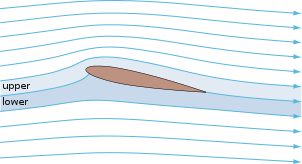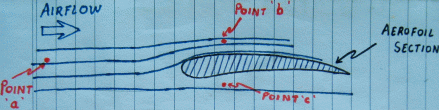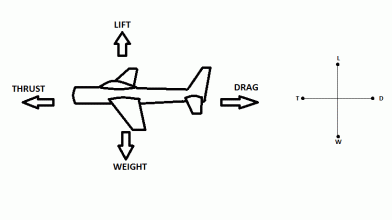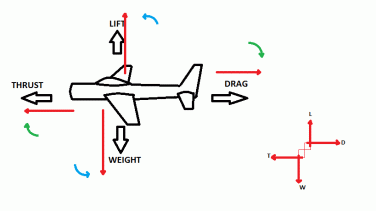•http://facebook.com/
•https://www.google.com/accounts/o8/id
•https://me.yahoo.com

# Basic Aerodynamics Theory

Understanding how an aeroplane derives lift with the Bernoulli's equation, and looking at the forces acting on an aeroplane in flight.

## Introduction

### Key Facts

Definitions

Law of Conservation of Energy Energy can neither be created, nor destroyed. It can only be said to change its state from one to another. As such, the total energy in any given system is always a constant.

Bernoullis principle
For a perfect incompressible liquid, flowing in a continuous stream, the total energy of a particle remains the same, while the particle moves from one point to another.

Kutta condition
A body with a sharp trailing edge which is moving through a fluid will create about itself a circulation of sufficient strength to hold the rear stagnation point at the trailing edge.

Lift
It is the component of force acting upwards and perpendicular to the line of flight, in a steady, undisturbed stream of air. It is important to note that 'upwards' implies to the roof of the aircraft in all attitudes of flight, i.e. Upright, Banked (as during a turn), and Inverted.
Aero-dynamics is the study of the dynamics associated with the motions of air over a moving object. When an aircraft accelerates down the runway to take off, it produces a relative motion between the air and the aircrafts wings. It is this rush of airflow over and below the wings, created by the aircrafts forward motion, which produces lift and consequently makes all forms of powered flight possible.Diagram 1 An aerofoil in a steady, streamlined, and incompressible flow of air. Image Courtesy http://en.wikipedia.org/wiki/Airfoil The contours of the upper and lower surfaces of the wing contribute largely to the pressure differences that occur between the two surfaces. The resulting pressure leads to a net force that provides 'Lift' to the wing.

In this section, we use the Bernoulli's and Euler's equations to understand how an aeroplane derives lift. We also look at the four principle forces acting on an aircraft in flight, at ALL times.

## Airflow Over An Aerofoil

### Bernoulli's Equation

As with all applications of the Bernoulli's principle, we shall assume that the flow of air around the aerofoil is:

• Incompressible, and
• Frictionless.Diagram 2 Aerofoil section in a Wind Tunnel experiment. Bernoulii's equation, considering the two points '' and '' in the airflow (refer Diagram 2), is given by:

where,
• and is the Pressure of the fluid at Point and respectively
• and is the Velocity of the fluid at Point and respectively
• and is the vertcal elevation at Point and respectively
• is the Density of the fluid
• is the acceleration due to gravity

For our calculation, we shall consider the values of and to be constant at both points and . We shall also assume the values of and to be negligible in a wind tunnel experiment. The equation can then be written as:

Since is at a point in the airflow that is unaffected by the passage of the aerofoil through the air, the values of pressure and velocity at this point can be considered to be the same as that of ambient air.Diagram 3 Aerofoil section 2 in a Wind Tunnel experiment. Now consider a Point (Diagram 3 above ) that is located below the aerofoil section.
Using Bernoulli's equation from (1), equating the values of pressure and velocity at this point () with those of ambient air, we get:

From Eq (2) and (3) we have:

According to the Kutta condition of circulation, the airflow over the upper surface of the aerofoil is faster than that below the underside of the aerofoil.
i.e.

Therefore, to satisfy the condition given in Eq(4),

The net result is an upward acting force which is termed as 'Lift'.

## Forces Acting In Flight

In the following diagram, we see four forces acting on an aircraft at all times.Diagram 4 Four Forces in Flight When an aircraft is in level flight, all the four fources are balanced and the aircraft can be said to be in a state of Equilibrium.
Lift() = Weight(), and
Thrust() = Drag()
In such state the aircraft will be travelling at a steady height, at a uniform velocity, and fixed heading.

### Climbing And Descending

The following discussion explains the relationship between these four forces when an aircraft is in the phase of gaining altitude (climbing), and when the aircraft descends.Forces during a Climb In the above diagram, consider the angle of climb to be .

Resolving the vectors about , we get:
• component directly opposing Thrust ; and,
• component directly opposing Lift .

Therefore, Implying that in a climb,

Also, Implying that
• (It is important to note the definition of Lift in this context)Forces during a Glide or Controlled Descent In the above diagram, consider the angle of descent to be .

Resolving the vectors we find that the Total Force, acting equal and opposite to the weight (), is a resultant of the Lift and Drag vectors. That is, This implies that
Or,

Higher the value of the ratio , the flatter the gliding angle - the more appropriate (for man and machinery) the descent from altitude.

Note : As we shall see in a later section, the ratio of L and D also has a significant effect on the Range and the Endurance(in other words the Performance) of the aircraft.

Note regarding Numericals In working out numericals associated with the Forces in Flight, it is important to note that the vectors (, , , ) are merely representative, and do not indicate their actual position. This is further explained in the diagram below.The Two Opposing Couples In low wing aircrafts, the vector is often located ahead of the vector; providing a couple with a nose-down pitching moment. Similarly, and directly opposing this (-) couple, the vector is located below the vector; providing a couple with a nose-up pitching moment.

Note: In the event of loss of power (),the nose-down pitching moment takes precedence, allowing the aircraft to enter into a glide. If a nose-up pitching moment was provided in such an event, the aircraft would inevitably stall and descend uncontrollably earthward. Thus the need for Weight and Balance of an aircraft, and the need to ensure that the C of G is within limits. This will be further analysed in future pages.

In addition,the forces acting on the Mainplane (Wings) are balanced by similar forces acting on the Tailplane (Stabilizer). All such numericals can be solved withe reference to the law of moments.

Example:
##### Example - Level Flight - With No Tail Plane Load
Problem
A seaplane of mass 20000 Kg. is flying in Straight and Level Flight. The thrust vector is located 1.2m ABOVE the drag vector. If the Lift/Drag ratio is 5:1, calculate the position of the lift vector. Consider the forces on the tail plane to be absent or negligable. Assume
Workings
By resolving the vectors it can be seen that in this case the Thrust and drag vectors produce a nose-down pitching moment. To oppose this, the Lift and Weight vectors will produce a nose-up pitching moment, i.e. Lift vector ahead of weight.

Let be the moment arm between and In st. and level flight we know,

= = 20000g kN

ratio = 5:1

Therefore, = 4000g kN =

Resolving the moments we get,

Solution
= 0.24m

The Lift vector is positioned 0.24m ahead of the Weight vector.